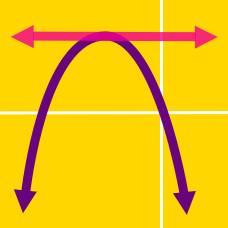Algebra

# Completing the Square: Level 5 Challenges

$16x^{4} + 8x^{3} - 12x^{2} - 4x +1 =0$ If $a,b,c$ and $d$ are the real roots of the above equation such that $a, then find the value of $\left\lfloor \dfrac{100ab}{cd} \right\rfloor$.

As $x, y, z$ ranges over all possible real numbers, what is the minimum value of

$3x^2+12y^2+27z^2-4xy-12yz-6xz-8y-24z+100 ?$

This problem has been proposed by Hui.An ambitious king plans to build a number of new cities in the wilderness, connected by a network of roads, so that any city can be reached from any other. He expects the annual tax revenues from each city to be numerically equal to the square of the population. Road maintenance will be expensive, though; the annual cost for each road is expected to be numerically equal to the product of the populations of the two cities that road connects. The project is considered viable as long as the tax revenues exceed the cost, regardless of the populations of the various cities (as long as at least one city has any inhabitants at all). The court engineer submits some proposals (attached), but the king deems them "boring" and asks for other options. How many other graphs are there (up to isomorphism) that make this project viable?

$\begin{cases}x^4+2x^3-y=-\frac{1}{4}+\sqrt{3}\\ y^4+2y^3-x=-\frac{1}{4}-\sqrt{3} \end{cases}$

All the ordered pairs of real numbers that satisfy the system of equations above are $(x_1,y_1),(x_2,y_2),...(x_n,y_n)$. Find the value of $x_1+x_2+...+x_n+y_1+...y_n$ correct upto two decimal places.

• If you think there are infinite solutions then answer 777 and if you think no real solutions answer 666.
• Ordered pair means (11,12),(12,11) are considered different.$P(x)=(x^2+5000x-1)^2+(2x+5000)^2$

Let $P(x)$ be a polynomial, then find the sum of all the real solutions to the equation $P(x)=\text{min}(P(x)).$

###### Image Credit: Wikimedia Five Thousand
×

Problem Loading...

Note Loading...

Set Loading...